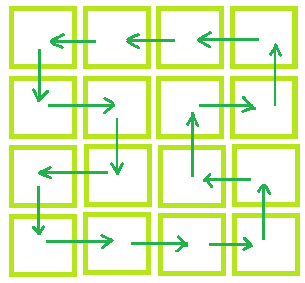# Check if possible to shuffle a matrix with adjacent movements

Given a N x M matrix which represents the number of rows and number of columns respectively. Each cell of a matrix is occupied by exactly one student. The task is to determine whether we can shuffle each student in such a way that each student should occupy a cell that is adjacent to that student’s original cell, i.e. immediately to the left, right, top or bottom of that cell and after shuffling each cell should occupy by exactly one student.

Examples:

Input: N = 3, M = 3
Output: Shuffling not possible

Input: N = 4, M = 4
Output: Shuffing is possible

One possible way to shuffle the student is as shown below:## Recommended: Please try your approach on {IDE} first, before moving on to the solution.

Approach: Check if the number of rows or the number of columns is even then shuffling is possible otherwise no shuffling is possible.

Below is the implementation of the above approach:

## C++

 `// C++ implementation of above approach ` `#include ` `using` `namespace` `std; ` ` `  `// Function that will check whether ` `// Shuffling is possible or not ` `void` `Shuffling(``int` `N, ``int` `M) ` `{ ` `    ``if` `(N % 2 == 0 || M % 2 == 0) ` `        ``cout << ``"Shuffling is possible"``; ` `    ``else` `        ``cout << ``"Shuffling not possible"``; ` `} ` ` `  `// Driver code ` `int` `main() ` `{ ` `    ``int` `N = 4, M = 5; ` ` `  `    ``// Calling function. ` `    ``Shuffling(N, M); ` `    ``return` `0; ` `} `

## Java

 `// Java implementation of above approach ` `import` `java.io.*; ` ` `  `class` `GFG  ` `{ ` `     `  `// Function that will check whether ` `// Shuffling is possible or not ` `static` `void` `Shuffling(``int` `N, ``int` `M) ` `{ ` `    ``if` `(N % ``2` `== ``0` `|| M % ``2` `== ``0``) ` `        ``System.out.println(``"Shuffling is possible"``); ` `    ``else` `        ``System.out.println(``"Shuffling not possible"``); ` `} ` ` `  `    ``// Driver code ` `    ``public` `static` `void` `main (String[] args)  ` `    ``{ ` `        ``int` `N = ``4``, M = ``5``; ` ` `  `    ``// Calling function. ` `    ``Shuffling(N, M); ` `    ``} ` `} ` ` `  `// This code is contributed by anuj_67.. `

## Python3

 `# Python3 implementation of above approach  ` ` `  `# Function that will check whether  ` `# Shuffling is possible or not  ` `def` `Shuffling(N, M) : ` `     `  `    ``if` `(N ``%` `2` `=``=` `0` `or` `M ``%` `2` `=``=` `0``) : ` `        ``print``(``"Shuffling is possible"``);  ` `    ``else` `: ` `        ``print``(``"Shuffling not possible"``);  ` ` `  `# Driver Code ` `if` `__name__ ``=``=` `"__main__"` `: ` ` `  `    ``# Driver code  ` `    ``N ``=` `4``;  ` `    ``M ``=` `5``;  ` ` `  `    ``# Calling function.  ` `    ``Shuffling(N, M);  ` `     `  `# This code is contributed by Ryuga `

## C#

 `// C# implementation of above approach ` `using` `System; ` ` `  `class` `GFG  ` `{ ` `     `  `// Function that will check whether ` `// Shuffling is possible or not ` `static` `void` `Shuffling(``int` `N, ``int` `M) ` `{ ` `    ``if` `(N % 2 == 0 || M % 2 == 0) ` `        ``Console.Write(``"Shuffling is possible"``); ` `    ``else` `        ``Console.Write(``"Shuffling not possible"``); ` `} ` ` `  `    ``// Driver code ` `    ``public` `static` `void` `Main ()  ` `    ``{ ` `        ``int` `N = 4, M = 5; ` ` `  `        ``// Calling function. ` `        ``Shuffling(N, M); ` `    ``} ` `} ` ` `  `// This code is contributed by Ita_c. `

## PHP

 ` `

Output:

```Shuffling is possible
```

My Personal Notes arrow_drop_upCheck out this Author's contributed articles.

If you like GeeksforGeeks and would like to contribute, you can also write an article using contribute.geeksforgeeks.org or mail your article to contribute@geeksforgeeks.org. See your article appearing on the GeeksforGeeks main page and help other Geeks.

Please Improve this article if you find anything incorrect by clicking on the "Improve Article" button below.

Article Tags :
Practice Tags :

Be the First to upvote.

Please write to us at contribute@geeksforgeeks.org to report any issue with the above content.